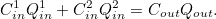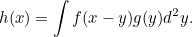## Influential Mathematicians: Gauss (3)

### Probability and Statistics

Gauss introduced what is now known as the Gaussian distribution: he showed how probability can be represented by a bell-shaped curve, with peaks around the mean when falls off quickly towards plus or minus infinity.

He also created the Gaussian function: a function of the formfor arbitrary real constants a, b and c.

### Modular Arithmetic

The modern approach to modular arithmetic was developed by Gauss in his book Disquisitiones Arithmeticae, published in 1801.  This now has application in number theory, abstract algebra, computer science, cryptography, and even in visual and musical art.

### Surveying

Whilst doing a surveying job for the Royal House of Hanover in the years after 1818, Gauss was also looking into the shape of the Earth and started to question what the shape of space itself was. This led him to question Euclidean geometry – one of the central tenets of the whole mathematics, which premised a flat universe, rather than a curved one. He later claimed that as early as 1800 he had already started to consider types of non-Euclidean geometry (where the parallel axiom does not hold), which were consistent and free of contradiction. However, to avoid controversy, he did not publish anything in this area and left the field open to Bolyai and Lobachevsky, although he is still considered by some to be the pioneer of non-Euclidean geometry.

This survey work also fuelled Gauss’ interest in differential geometry, which uses differential calculus to study problems in geometry involving curves and surfaces. He developed what has become known as Gaussian curvature. This is an intrinsic measure of curvature that depends only on how distances are measured on the surface, not on the way it is embedded in space.

His achievements during these years, however, was not only limited to pure mathematics. He invented the heliotrope, which is an instrument that uses a mirror to reflect sunlight over great distances to mark positions in a land survey.

All in all, this period of time was one of the most fruitful periods of his academic life; he published over 70 papers between 1820 and 1830.

In later years, he worked with Wilhelm Weber to make measurements of the Earth’s magnetic field, and invented the first electric telegraph.

Read part 1 here and part 2 here.

Let me know what you think of this new series! M x

## MATHS BITE: Stars and Bars

A common problem in combinatorics is when we are asked to count the number of ways to group identical objects, such as placing indistinguishable balls into labelled urns. Popularised by William Feller in his book on probability, the Stars and Bars methods aims to solve such problems.

### Theorem

The number of ways to put n indistinguishable balls into k labelled urns isProof using Stars and Bars:

Represent n balls by n adjacent stars and consider inserting k – 1 bars between the starts to separate them into k groups.

For example, for n = 12 and k = 5 the following is a possible arrangement:

**|****||***|***

Here, urns 1, 2, 3, 4 and 5 are of size 2, 4, 0, 3 and 3 respectively.

There are a total of n + k – 1 positions, of which n are stars and k – 1  are bars. So, the number of ways to place n indistinguishable balls into k labeled urns in the same as the number of ways of choosing n positions (or k – 1 positions) amount n + k – 1 spaces, with all the remaining positions taken as bars (stars), i.e.ways.

M x

## Markov Chains: An Intro

### The Markov Property

A stochastic process (“a mathematical object usually defined as a collection of random variables”) is said to have the Markov Property if, conditional on the present value, the future is independent on the past.

Let’s firstly introduce some notation: let S be a countable set called the state space and let = (Xt: t ≥ 0) be a sequence of random variables taking values in S.

Then, the sequence X is called a Markov Chain if it satisfies the Markov Property:for all t ≥ 0 and all x0, x1, …, xt ϵ S.

Notation is simplified in the case where the Markov chain is homogeneous. This is when for all i, j ϵ S, the conditional probability P(Xt+1 = j | Xt = i) does not depend on the value of t.

### Examples

• Branching Process: The branching process is a simple model of the growth of a population; each member of the nth generation has a number of offspring that is independent of the past; Xn = size of the nth generation.
• Random Walk: A particle performs a random walk on the line: let Z1, Z2, …, be independent with P(Zi = 1) = p and P(Zi = -1) = 1-p, then Xn =
Z1 + … + Zn; at each epoch of time, it jumps a random distance that is independent of the previous jumps.
• Poisson Process: the Poisson process satisfies a Markov property in which time is a continuous variable rather than a discrete variable, and thus the Poisson process is an example of a continuous-time Markov chain; the Markov property still holds as arrivals after time t are independent of arrivals before this time.

### Two Quantities

For simplification (and as this is only an intro to  Markov chains) we’ll assume that the Markov chains are homogeneous.

Two quantities that are needed in order to calculate the probabilities in a chain are the:

1. transition matrix: P = (pi,j: i,j ϵ S) given by pi,j = P(X1 = j | X0 = i);
2. initial distribution: λ = (λi : i ϵ S) given by λi = P(X0 = i).

As we have assumed homogeneity we have that

pi,j = P(Xn+1 = j | Xn = i) for n ≥ 0.

These quantities are characterised in the following way:

#### Proposition:

a) The vector λ is a distribution  in that λi ≥ 0 for i ϵ S and the sum of λi over i = 1.

b) The matrix P = (pi,j) is a stochastic matrix in that pi,j ≥ 0 for i, j ϵ S and the sum of pi,j over j = 1 for i ϵ S (i.e. that P row sums to 1)

#### Proof:

a) As λi is a probability, it is clearly non-negative. Additionally, the sum of λi over i = the sum of P(X0 = i) over i = 1.

b) Since pi,j is a probability, it is non-negative. Finally, the sum of pi,j over j = the sum of P(Xn+1 = j | Xn = i) over j = P(Xn+1 ϵ S | Xn = i) = 1.

Hope you enjoyed this brief introduction to a Markov Chain!

M x

## Seven Statistical Sins

Inspired by an article on phys.org I decided to compile a list of the seven statistical sins. Statistics is a vital tool to understanding the patterns in the world around us, however our intuition often lets us down when it comes to interpreting these patterns.

1.Assuming small differences are meaningful

Examples of this include small fluctuations in the stock market, or differences in polls where one party is ahead by one point or two. These represent chance rather than anything meaningful.

To avoid drawing any false conclusions that may arise due to this statistical noise we must consider the margin of error related to the numbers. If the difference is smaller than the margin of error, there is likely no meaningful difference and is probably due to random fluctuations.

2. Equating statistical significance to real-world significance

Statistical data may not represent real-world generalisations, for example stereotypically women are more nurturing while men are physically stronger. However, given a pile of data, if you were to pick two men at random there is likely to be quite a lot of difference in their physical strength; if you pick one man and one women they may end up being very similar in terms of nurturing or the man may be more nurturing than the woman.

This error can be avoided by analysing the effect size of the differences between groups, which is a measure of how the average of one group differs from the average of another. Then if the effect size is small, the two groups are very similar. Even if the effect size is large, each group will still have a lot of variation so not all members of one group will be different from all members of the other (hence giving rise to the error described above).

3. Neglecting to look at the extremes

This is relevant when looking at normal distributions.

In these cases, when there is a small change in performance for the group, whilst there is no effect on the average person the character of the extremes changes more drastically. To avoid this, we have to reflect on whether we’re dealing with the extreme cases or not. If we are, these small differences can radically affect the data.

4. Trusting coincidence

If we look hard enough, we can find patterns and correlations between the strangest things, which may be merely due to coincidence. So, when analysing data we have to ask ourselves how reliable the observed association is. Is it a one-off? Can future associations be predicted? If it has only been seen once, then it is probably only due to chance.

5. Getting causation backwards

When we find a correlation between two things, for example unemployment and mental health, it may be tempting to see a causal path in one direction: mental health problems lead to unemployment. However, sometimes the causal path goes in the other direction: unemployment leads to mental health problems.

To get the direction of the causal path correct, think about reverse causality when you see an association. Could it go in the other direction? Could it even go in both ways (called a feedback loop)?

6. Forgetting outside cases

Failure to consider a third factor that may create an association between two things may lead to an incorrect conclusion. For example, there may be an association between eating at restaurants and high cardiovascular strength. However, this may be due to the fact that those who can afford to eat at restaurants regularly are in high socioeconomic bracket, which in turn means they can also afford better health care.

Therefore, it is crucial to think about possible third factors when you observe a correlation.

7. Deceptive Graphs

A lot of deception can arise from the way that the axis are labeled (specifically the vertical axis) on graphs. The labels should show a meaningful range for the data given. For example, by choosing a narrower range a small difference looks more impactful (and vice versa).

In fact, check out this blog filled with bad graphs.

M x

## Stirling’s Formula

Today I wanted to discuss something I learnt last week in my Probability course: Stirling’s Formula. Stirling’s Formula is an approximation for factorials, and leads to quite accurate results even for small values of n.

The formula can be written in two ways:orwhere the ~ sign means that the two quantities are asymptotic (i.e. their ratios tend to 1 as n tends to infinity).

## Proof of Stirling’s Formula

The following identity arises using integration by parts:Taking f(x) = log x, we obtainNext, sum over n, and by recalling that log x + log y = log xy we get the following expression:whereNext, definewhich allows us to rearrange the above expression to:So as n tends to infinity we get

How do we show that?

Firstly, note that from (*) it follows thatSo, we need to show thatLet’s setNote that I0=π/2 and I1 = 1. Then for n≥2, we can integrate by parts to see thatAnd so, we obtain the following two expressions:In is decreasing in n and In/In-2 → 1, so it follows that I2n/I2n+1 → 1. Therefore,as required.

Although the end result is satisfying, I find that some steps in this proof are like ‘pulling-a-rabbit-out-of-a-hat’! What do you think? Mx

## Probability: Equally Likely Outcomes

Starting a new term in university comes with 4 brand new topics to learn. One of these is Probability. I’d like to share a little bit of (simple) maths that I learnt in my lecture last week on calculating probabilities when there are equally likely outcomes.

I will not define what a probability measure or space is rigorously, as it is quite irrelevant for the simple examples I’m considering today. But, I will introduce the following notation:

Let Ω be a set and F be a set of all subsets of Ω. The elements of Ω are called outcomes and the elements of F are called events. As we are using this as a probability model, Ω will be an abstraction of a real set of outcomes, and F will model observable events.

Then let us write, P(A) as the probability of the event A occurring. Note that:

P(A) = |A|/|Ω|.

Now, let us consider a few examples of how we would use this. In the following examples we use symmetry and randomness to argue that there are equally likely outcomes.

### Throwing a Die

The throw of a die has six possible outcomes, and so

Ω = {1, 2, 3, 4, 5, 6} and P(A) = |A|/6 for A ⊆ Ω.

Therefore, for example P({2, 4, 6}) = 1/2.

### Balls from a Bag

A bag contains r balls. Say we draw k balls from this bag without looking. If we consider that the balls are labelled by {1, . . . , n}, we have selected a subset of {1, . . . , n} of size k.

Therefore, Ω is the set of subsets of {1, . . . , n} of size k, sowith the probability of a individual outcome being 1/|Ω|.

### Pack of Cards

In an idealised well-shuffled pack of cards, every possible order is equally likely, so it is modelled by the set Ω of permutations of {1, . . . , 52}.

Because of this, we can see that |Ω| = 52!

What is the probability of the event A that the first two cards are aces?

There are 4 choices for the first ace, 3 choices for the second, and the rest can come in any order. So,

|A| = 4 × 3 × 50!

and

P(A) = |A|/|Ω| = 12/(52 × 51) = 1/221.

### Distribution of Largest Digit

Suppose that r digits are chosen from a table of random numbers. What is the probability that k is the greatest digit drawn?

Firstly, model the set of possible outcomes by

Ω = {0, 1, . . . , 9}n

Consider the event Ak = [no digit exceeds k], and the event Bk  = [the largest digit is k] So:

|Ω| = 10n ;

|Ak| = (k + 1)n ;

|Bk| = |Ak \ Ak−1| = (k + 1)n − kn.

Therefore, P(Bk) = [(k + 1)n − kn]/10n.

Hope you enjoyed today’s post. M x

## Maths on Trial

Last week I had the pleasure to attend a talk by Leila Schneps on the mathematics of crime. A self-declared pure mathematician, Schneps recently became involved in studying the mathematics in criminal trials. Rather than focusing on the mathematics of forensic data, such as DNA, the talk was on the use of Bayesian networks in crime evidence analysis.

One of the most challenging tasks for juries in criminal trials is to weigh the impact of different pieces of evidence which may be dependent on each other. In fact, studies have shown that there are several fallacies that jury members fall into on a regular basis, such as overestimating the weight of a single piece of DNA evidence. This is because most people assume that the probability of an event A happening given that B happens [P(A|B)] is equal to an event B happening, given that A happens [P(B|A)]. However, this is NOT true: the two are connected by Bayes’ Rule.For example, a forensic specialist may say that a piece of DNA is found in 1 in every 1000 people. The jury will take this to mean the the suspect must be guilty as he is that person out of 1000 (the chances of it being anyone else is so low). However, this is critically not true, as explained above.

Thus, Bayesian networks are a powerful tool to assess the weight of different kinds of evidence, taking into account their dependencies on one another, and what effect they have on the guilt of the suspect.

What are Bayesian Networks?

Bayesian Networks are a type of statistical model,  which organise a body of knowledge in any given area, in this case evidence of a criminal trial, by mapping out cause-and-effect relationships and encoding them with numbers that represent the extent to which one variable is likely to affect the other. These networks are named after British mathematician Reverend Thomas Bayes, due to their reliance on Bayes’ formula. This rule can be extended to multiple variables and multiple states, allowing complicated probabilities to be calculated.

Example

To illustrate an easy example, consider this Bayesian Network:Let us say that the weather can only have three states: sunny, cloudy or rainy, that the grass can only be wet or dry, and that the sprinkler can be on or off. The arrows, which represent dependence, have been drawn in that way because if it rainy, then the lawn will be wet, but if it is sunny for a long time then this will cause us to turn on the sprinklers, and hence the lawn will also be wet.

By imputing probabilities into this network that reflect the reality of real weather, lawn and sprinkler-use behaviour, it can be made to answer questions like:

“If the lawn is wet, what are the chances it was caused by rain or by the sprinkler?”

“If the chance of rain increases, how does that affect my having to budget time for watering the lawn?”

In her presentation, Leila Schneps talked briefly about a book she had released entitled ‘Math on Trial’, which describes 10 trials spanning the 19th century to today, in which mathematical arguments were used (and greatly misused) as evidence. The cases discussed include “Sally Clark, who was accused of murdering her children by a doctor with a faulty sense of calculation; of nineteenth-century tycoon Hetty Green, whose dispute over her aunt’s will became a signal case in the forensic use of mathematics; and of the case of Amanda Knox, in which a judge’s misunderstanding of probability led him to discount critical evidence – which might have kept her in jail.”

After hearing Schneps transmit her passion and excitement, I am fascinated with this subject and can’t wait to get my hands on this book to learn more! M x

## Benford’s Law

Benford’s Law is names after the American physicist Frank Benford who described it in 1938, although it had been previously mentioned by Simon Newcomb in 1881.

Benford’s Law states that in “naturally occurring collections of numbers” the leading significant digit is more likely to be small. For example, in sets of numbers which obey this law, the number 1 appears as the first significant digit about 30% of the time, which is much greater than if the digits were distributed uniformly: 11.1%.

In mathematics, a set of numbers satisfies this law if the leading digit, d, occurs with a probability:Hence, if d = 1, then P(1) = log 2 = 30.1..%

The leading digits have the following distribution in Benford’s law:

As P(d) is proportional to the space between d and d+1 on a logarithmic scale, the mantissae of the logarithms of the numbers are uniformly and randomly distributed.

## Applications

Benford’s law has found applications in a big variety of data sets, such as stock prices, house prices, death rates and mathematical constants.

Due to this, fraud can be found applying Benford’s law to data sets. This is because if a person is trying to fabricate ‘random’ values to try to not appear suspicious, they will probably select numbers such that the initial digits are uniformly distributed. However, as explained above, this is completely wrong! In fact, this application of Benford’s law is so powerful that there is an “industry specialising in forensic accounting and auditing which uses these phenomena to look for inconsistencies in data.”

Datagenetics.com describes how:

“In 1993, in State of Arizona v. Wayne James Nelson (CV92-18841), the accused was found guilty of trying to defraud the state of nearly $2 million, by diverting funds to a bogus vendor. The perpetrator selected payments with the intention of making them appear random: None of the check amounts was duplicated, there were no round-numbers, and all the values included dollars and cents amounts. However, as Benford’s Law is esoterically counterintuitive, he did not realize that his seemingly random looking selections were far from random.” Sources: 1 | 2 | 3 | 4 After writing this I’ve realised that I touched on this law (in less detail) in a previous post during my Christmas series! M x ## St. Petersburg Paradox The St. Petersburg Paradox is a problem related to probability and decision theory in economics. It’s based on a lottery game that results in a random variable with infinite expected value, but still seems to be worth only a small amount to the players. The paradox is as follows: “A casino offers a game of chance for a single player in which a fair coin is tossed at each stage. The pot starts at 2 dollars and is doubled every time a head appears. The first time a tail appears, the game ends and the player wins whatever is in the pot. Thus the player wins 2 dollars if a tail appears on the first toss, 4 dollars if a head appears on the first toss and a tail on the second, and so on. Expressing this mathematically, the player wins 2k dollars, where k equals number of tosses and is a positive integer. What would be a fair price to pay the casino for entering the game?” In order to answer this we must consider the average payout. As the probability of getting 2 dollars is 1/2,the probability of getting 4 dollars is 1/4 and so on, the expected value is:Note that we must assume that the game can continue infinitely, i.e. the casino has unlimited resources, hence the sum grows without bound. Thus, as the expected value is infinite, one should play the game at any price if offered the opportunity. However, as described by Ian Hacking “few of us would pay even$25 to enter”. The paradox arises from the difference in what people are willing to pay in real life, versus the infinite expected value.

### Expected Utility?

To Daniel Bernouilli, “the determination of the value of an item must not be based on the price, but rather on the utility it yields”, and hence suggested that the answer relied on the expected utility, rather than expected value. Bernoulli proposed a utility model which involves the logarithmic function U(w) = ln(w), where w is the gambler’s total wealth. Note that each possible event in weighted by the probability of that event happening. If c is the cost of entering the game, the expected incremental utility converges to a finite value:This formula gives a relationship between the gambler’s wealth and how much he should pay. For example, a millionaire should be willing to pay up to $20.88 and a person with$1,000 should pay up to \$10.95.

However, this solution was criticised by other economists. Alfred Marshall replaced ‘wealth’ in Bernoulli’s solution by ‘income’, which changed the main conclusions of the paradox. Marshall explains how, when assuming decreasing marginal utility, no rational individual would play the game since losses would be greater than gains.Consider the probability of loss being equal to the probability of winning = ½. If a player loses, they will lose the money paid to play the game (x-1) but if they win, the player will win exactly the amount paid (x+1). Since area A is greater than B no rational player would play.

Alfred Marshall puts it in such way:

“The clerk with £100 a-year will walk to business in a much heavier rain than the clerk with £300 a-year; for the cost of a ride by tram or omnibus measures a greater benefit to the poorer man than to the richer. If the poorer man spends the money, he will suffer more from the want of it afterwards than the richer would. The benefit that is measured in the poorer man’s mind by the cost is greater than that measured by it in the richer man’s mind.”

Hope you enjoyed today’s post! M x

## Fighting crime with Maths

I was inspired to write this post by a video I watched on Numberphile’s YouTube channel, which I would highly recommend watching as I found it extremely interesting.

To summarise, in the video Hannah Fry talks about how the frequency of crime events can be explained mathematically using the Poisson Distribution and Hawke’s process (which takes into account the fact that events aren’t completely independent from one another). As soon as we establish how frequent crime happens in certain places, this information can then be used to predict in what areas a robbery, for example, will most likely happen on a certain night, using formulas derived using these probability distributions.

This led me to research in what ways mathematics is helping to fight crime.

Mathematical Modelling

In UCLA, researchers were able to build a mathematics model that allows then to analyse different types of criminal ‘hotspots’ – places where many crimes occur. They found that there are two types of hotspots: Super-Critical Hotspots where there are small spikes in crime that grow and ‘Subcritical Hotspots’ generated by a large spike in crime that pulls offenders into a central location. This finding has allowed policing actions to be customised to the type of hotspot as their actions will be very different for each. Additionally, using their mathematical model, the scientists are able to predict how each type of hotspot will respond to increased policing, as well as when each type might occur using bifurcation theory.

Inverse Problems

Inverse problems are mathematical detective problems. To solve an inverse problem, mathematicians need a physical model of the event to understand what causes lead to what effects. Then, given the known effects, mathematics can be used to give the possible causes. Furthermore, maths is also used to establish the limitations of the model and therefore the accuracy of the answer. Examples of inverse problems include remote sensing of the lad or sea from satellite images, using medical images for diagnosing tumours and interpreting seismographs to prospect for oil.

Inverse problems are extremely relevant to solving crimes; evidence which is left at the a crime scene must be analysed and then scientists must work backwards to deduce what happened and who did it.

Speeding

Mechanics can be extremely useful when analysing evidence when vehicles are involved, for example when analysing skid marks. Skid marks are caused by the speed of the car as well as other factors such as braking force, friction with the road and impacts with other vehicles.  Mathematically, mechanics can be used to model this event: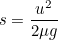: length of skid,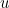: speed of the vehicle,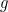: acceleration due to gravity and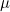: coefficient of friction times braking efficiency.

Say we wanted to know if the car was speeding, the equation can be rearranged to: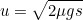allowing the speed of the car to be determined.

Contaminants

Mathematics can also be used to find where a contaminant was released by modelling how the water and contaminant flow through the water network. As the contaminant flows, its its concentration decreases. This decrease can be described by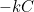.

Firstly,depends on time and this change in concentration as time passes is shown by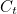. Secondly, it is also dependant on the contaminants reaction with the space it is in (in this case the pipe) which is influenced by the volume and flow of the water. Let’s writes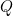for water flow and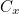for the changes in concentration due to the surrounding space, and from this we can create a mathematical model for the overall loss in concentration of the contaminant: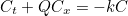Furthermore, we must take into account that at each junction different solutions mix together: